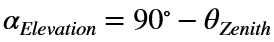# Problem 56323. Air Mass to Star for an Observer at Mean Sea Level

Air mass is a measure of how much atmosphere light from a source above the atmosphere (eg sun, planet, star) has to travel through to reach an observation point on the Earth surface. It’s measured as a ratio of the amount of atmosphere relative to the amount of atmosphere for an overhead (nadir) line-of-sight.
To calculate air mass, divide the value of 1 by the cosine of the zenith angle.The elevation angle of a star is given with respect to the local astronomical horizon:Write a function to calculate the Air Mass a star's light passes through from the line-of-sight elevation angle in degrees. Allow the function to compute air mass for an array of line-of-sight elevation angles.

### Solution Stats

24.93% Correct | 75.07% Incorrect
Last Solution submitted on Dec 08, 2023

### Community Treasure Hunt

Find the treasures in MATLAB Central and discover how the community can help you!

Start Hunting!